# 面试中的 HashMap、ConcurrentHashMap 和 Hashtable，你知道多少？ClericYi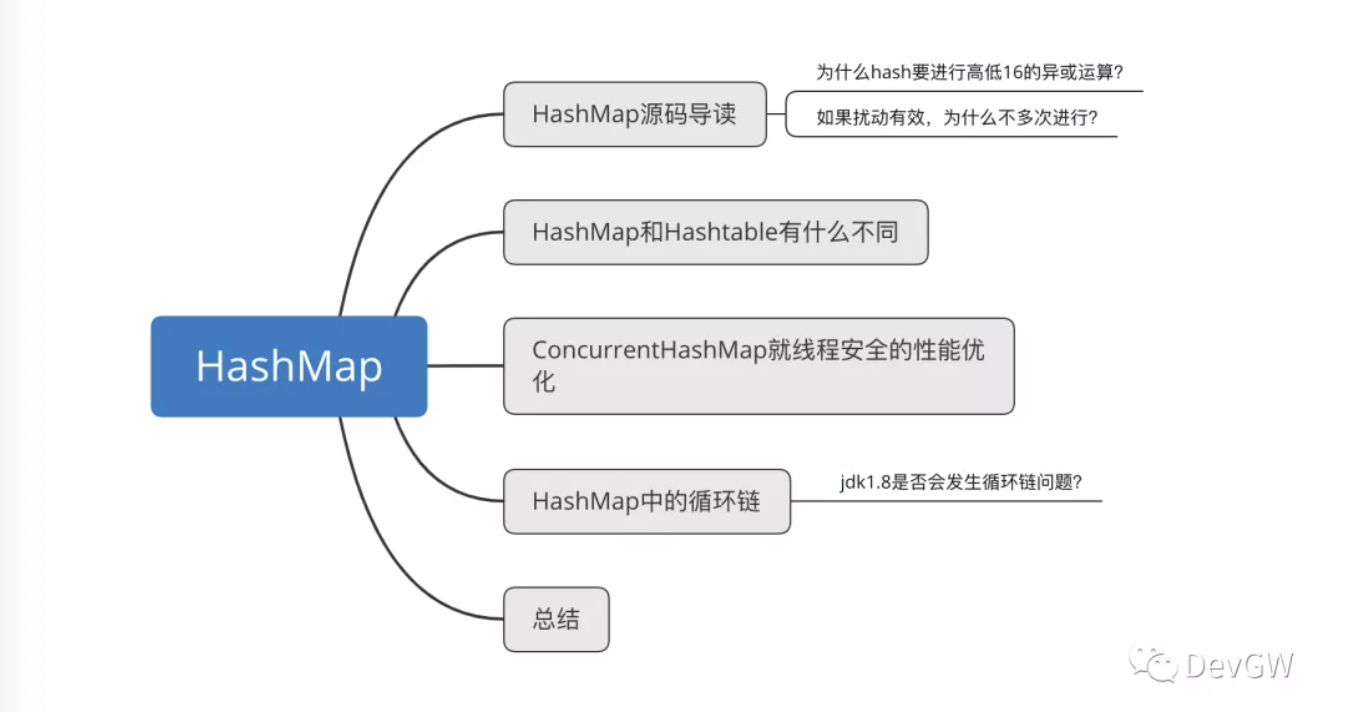# HashMap源码导读

HashMap hashMap = new HashMap();hashMap.put(key, value);hashMap.get(key);

## HashMap()

public HashMap(int initialCapacity, float loadFactor) {        if (initialCapacity < 0)            throw new IllegalArgumentException("Illegal initial capacity: " +                                               initialCapacity);        // 对数组的一个保护，不能超过int最大值范围        if (initialCapacity > MAXIMUM_CAPACITY)            initialCapacity = MAXIMUM_CAPACITY;        if (loadFactor <= 0 || Float.isNaN(loadFactor))            throw new IllegalArgumentException("Illegal load factor: " +                                               loadFactor);        this.loadFactor = loadFactor;        this.threshold = tableSizeFor(initialCapacity);    }    public HashMap(int initialCapacity) {        this(initialCapacity, DEFAULT_LOAD_FACTOR);    }    public HashMap() {        this.loadFactor = DEFAULT_LOAD_FACTOR; // all other fields defaulted    }    public HashMap(Map<? extends K, ? extends V> m) {        this.loadFactor = DEFAULT_LOAD_FACTOR;        putMapEntries(m, false);    }

static final int tableSizeFor(int cap) {        int n = cap - 1;        n |= n >>> 1;        n |= n >>> 2;        n |= n >>> 4;        n |= n >>> 8;        n |= n >>> 16;        return (n < 0) ? 1 : (n >= MAXIMUM_CAPACITY) ? MAXIMUM_CAPACITY : n + 1;    }

## put(key, value)

public V put(K key, V value) {        return putVal(hash(key), key, value, false, true); // 1    }// 由注释1直接调用的方法putVal()final V putVal(int hash, K key, V value, boolean onlyIfAbsent,                   boolean evict) {        Node<K,V>[] tab; Node<K,V> p; int n, i;        // 第一次来判断的时候，显然的tab是一个空，因为在构造函数中，我们并没有看到他的初始化，那么必然要调用resize()方法。        if ((tab = table) == null || (n = tab.length) == 0)            n = (tab = resize()).length; // 2，未能初始化而必然调用的方法        if ((p = tab[i = (n - 1) & hash]) == null)            tab[i] = newNode(hash, key, value, null);        else {            Node<K,V> e; K k;            if (p.hash == hash &&                ((k = p.key) == key || (key != null && key.equals(k))))                e = p;            else if (p instanceof TreeNode)                e = ((TreeNode<K,V>)p).putTreeVal(this, tab, hash, key, value);            else {                for (int binCount = 0; ; ++binCount) {                    if ((e = p.next) == null) {                        p.next = newNode(hash, key, value, null);                        if (binCount >= TREEIFY_THRESHOLD - 1) // -1 for 1st                            treeifyBin(tab, hash);                        break;                    }                    if (e.hash == hash &&                        ((k = e.key) == key || (key != null && key.equals(k))))                        break;                    p = e;                }            }            if (e != null) { // existing mapping for key                V oldValue = e.value;                if (!onlyIfAbsent || oldValue == null)                    e.value = value;                afterNodeAccess(e);                return oldValue;            }        }        ++modCount;        if (++size > threshold)            resize(); // 2        afterNodeInsertion(evict);        return null;    }// 由注释2直接调用的方法// 由多种方法调用到这里：// 1. 尚未初始化// 2. 保存的数据超出 容量 * 负载因子// 3. 数据被删的不足以支持树形的时候final Node<K,V>[] resize() {        Node<K,V>[] oldTab = table;        int oldCap = (oldTab == null) ? 0 : oldTab.length;        int oldThr = threshold;        int newCap, newThr = 0;        // 。。。。        // 此处对容量大小做了一系列的判定，为定义初始化容量为16        // 。。。。        if (newThr == 0) {            float ft = (float)newCap * loadFactor;            newThr = (newCap < MAXIMUM_CAPACITY && ft < (float)MAXIMUM_CAPACITY ?                      (int)ft : Integer.MAX_VALUE);        }        threshold = newThr;        // 进行了整个的table进行一个初始化        // 而这个table就是一个Node的数组        // Node也就是链表的一个个节点，读者自己点进去观察就能看到next节点        @SuppressWarnings({"rawtypes","unchecked"})        Node<K,V>[] newTab = (Node<K,V>[])new Node[newCap];        table = newTab;        // 。。。。。    }

## get(key)

public V get(Object key) {        Node<K,V> e;        return (e = getNode(hash(key), key)) == null ? null : e.value;    }

static final int hash(Object key) {        int h;        return (key == null) ? 0 : (h = key.hashCode()) ^ (h >>> 16);    }

final Node<K,V> getNode(int hash, Object key) {        Node<K,V>[] tab; Node<K,V> first, e; int n; K k;        if ((tab = table) != null && (n = tab.length) > 0 &&            (first = tab[(n - 1) & hash]) != null) {            if (first.hash == hash && // always check first node                ((k = first.key) == key || (key != null && key.equals(k))))                return first;            if ((e = first.next) != null) {                if (first instanceof TreeNode)                // 对树形中的数据进行查找                    return ((TreeNode<K,V>)first).getTreeNode(hash, key);                // 对链表中的数据进行查找                do {                    if (e.hash == hash &&                        ((k = e.key) == key || (key != null && key.equals(k))))                        return e;                } while ((e = e.next) != null);            }        }        return null;    }

## 为什么hash要进行高低16的异或运算？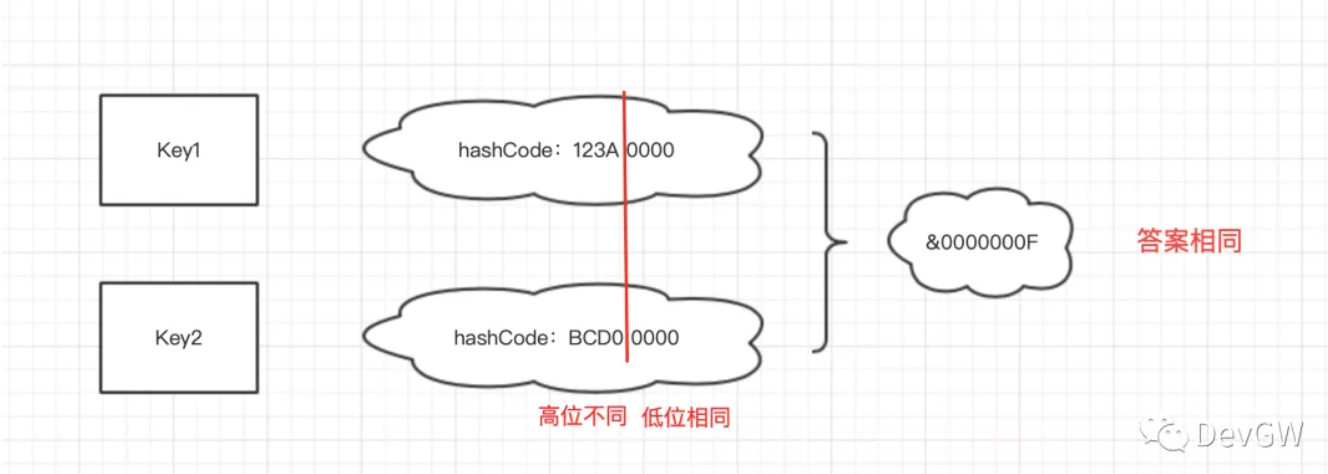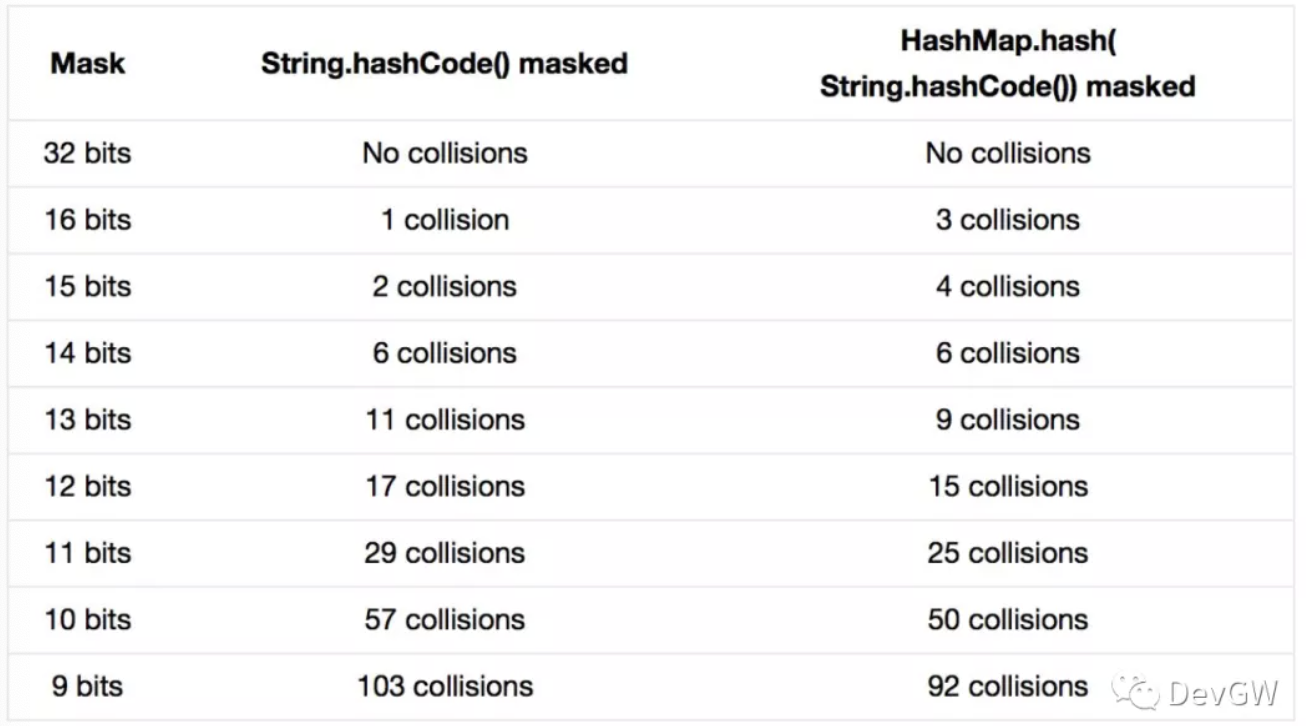# HashMap和Hashtable有什么不同

// 无参构造函数初始化，处理容量为11，负载因子为0，75public Hashtable() {        this(11, 0.75f);    }// 链表的创建在默认最后嗲用的构造函数中就已经创建// 那这里我们就发现了第一个不同的地方。public Hashtable(int initialCapacity, float loadFactor) {        if (initialCapacity < 0)            throw new IllegalArgumentException("Illegal Capacity: "+                                               initialCapacity);        if (loadFactor <= 0 || Float.isNaN(loadFactor))            throw new IllegalArgumentException("Illegal Load: "+loadFactor);        if (initialCapacity==0)            initialCapacity = 1;        this.loadFactor = loadFactor;        table = new Entry<?,?>[initialCapacity];        threshold = (int)Math.min(initialCapacity * loadFactor, MAX_ARRAY_SIZE + 1);    }

public synchronized V put(K key, V value) {        // 判空机制的存在，和HashMap并无判空，也就容许null作为key存在        if (value == null) {            throw new NullPointerException();        }        // Makes sure the key is not already in the hashtable.        Entry<?,?> tab[] = table;        int hash = key.hashCode();        int index = (hash & 0x7FFFFFFF) % tab.length;        @SuppressWarnings("unchecked")        Entry<K,V> entry = (Entry<K,V>)tab[index];        for(; entry != null ; entry = entry.next) {            if ((entry.hash == hash) && entry.key.equals(key)) {                V old = entry.value;                entry.value = value;                return old;            }        }        addEntry(hash, key, value, index);        return null;    }

private void addEntry(int hash, K key, V value, int index) {        modCount++;        Entry<?,?> tab[] = table;        if (count >= threshold) {            // Rehash the table if the threshold is exceeded            rehash();            tab = table;            hash = key.hashCode();            index = (hash & 0x7FFFFFFF) % tab.length;        }        // Creates the new entry.        @SuppressWarnings("unchecked")        Entry<K,V> e = (Entry<K,V>) tab[index];        tab[index] = new Entry<>(hash, key, value, e);        count++;    }

# ConcurrentHashMap就线程安全的性能优化

（1）和HashMap的实现方式一样，数组+链表+红黑树，查找性能上优于Hashtable前提： 使用的容量大于8。

（2）分段锁机制 / 锁槽机制：不再是整个数组加锁，而是对单条或者几条链表和红黑树进行加锁，也就同时能够就收多个不同的hash操作了。

final V putVal(K key, V value, boolean onlyIfAbsent) {        if (key == null || value == null) throw new NullPointerException();        int hash = spread(key.hashCode());        int binCount = 0;        for (Node<K,V>[] tab = table;;) {            Node<K,V> f; int n, i, fh;            if (tab == null || (n = tab.length) == 0)                //。。。。。            }            else if ((f = tabAt(tab, i = (n - 1) & hash)) == null) {                // 引入了CAS机制                if (casTabAt(tab, i, null,                             new Node<K,V>(hash, key, value, null)))                    break;                   // no lock when adding to empty bin            }            else if ((fh = f.hash) == MOVED)                //。。。。。            else {                V oldVal = null;                synchronized (f) {                    // 。。。。。                }                //。。。。。            }        }        addCount(1L, binCount);        return null;    }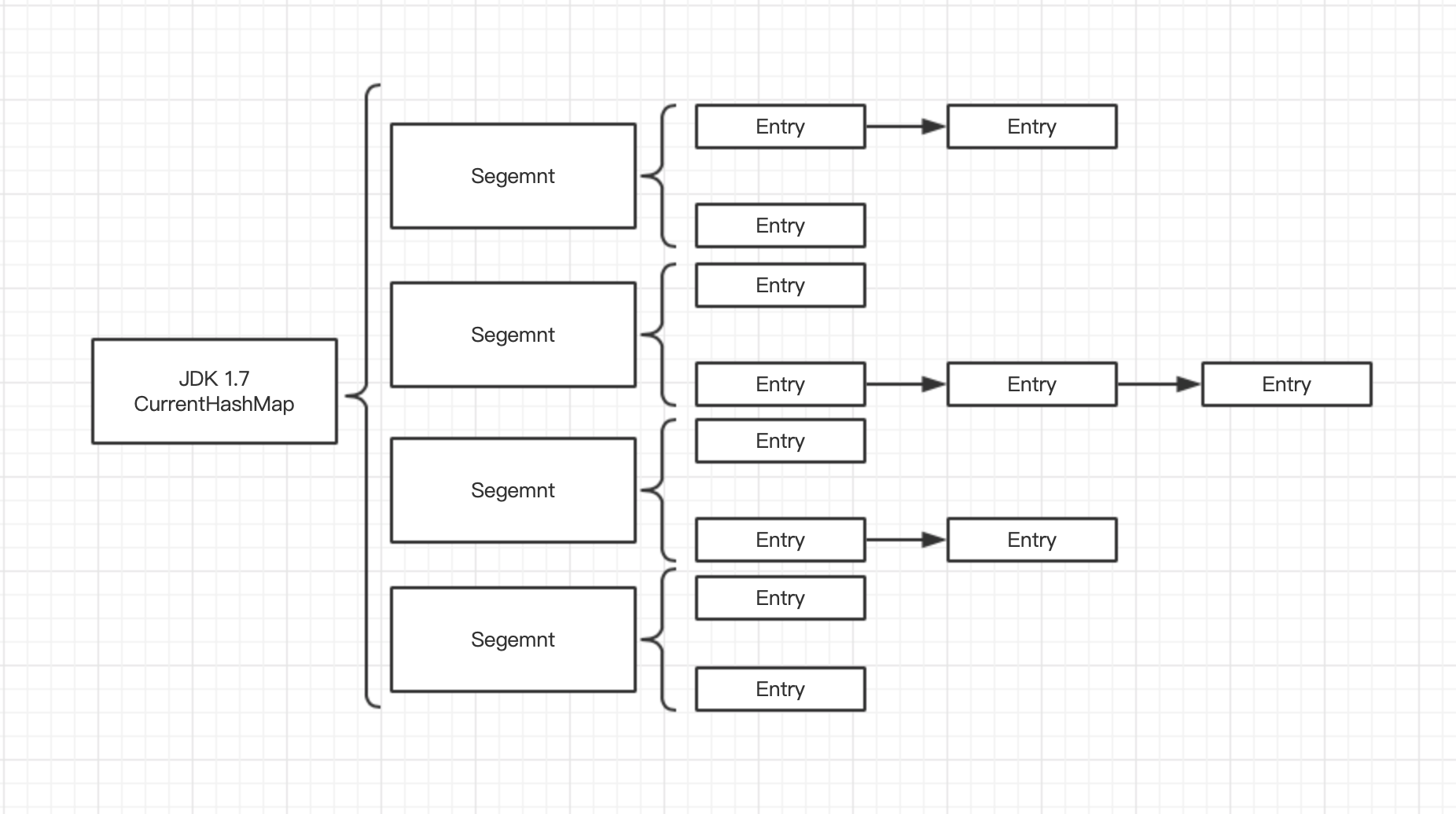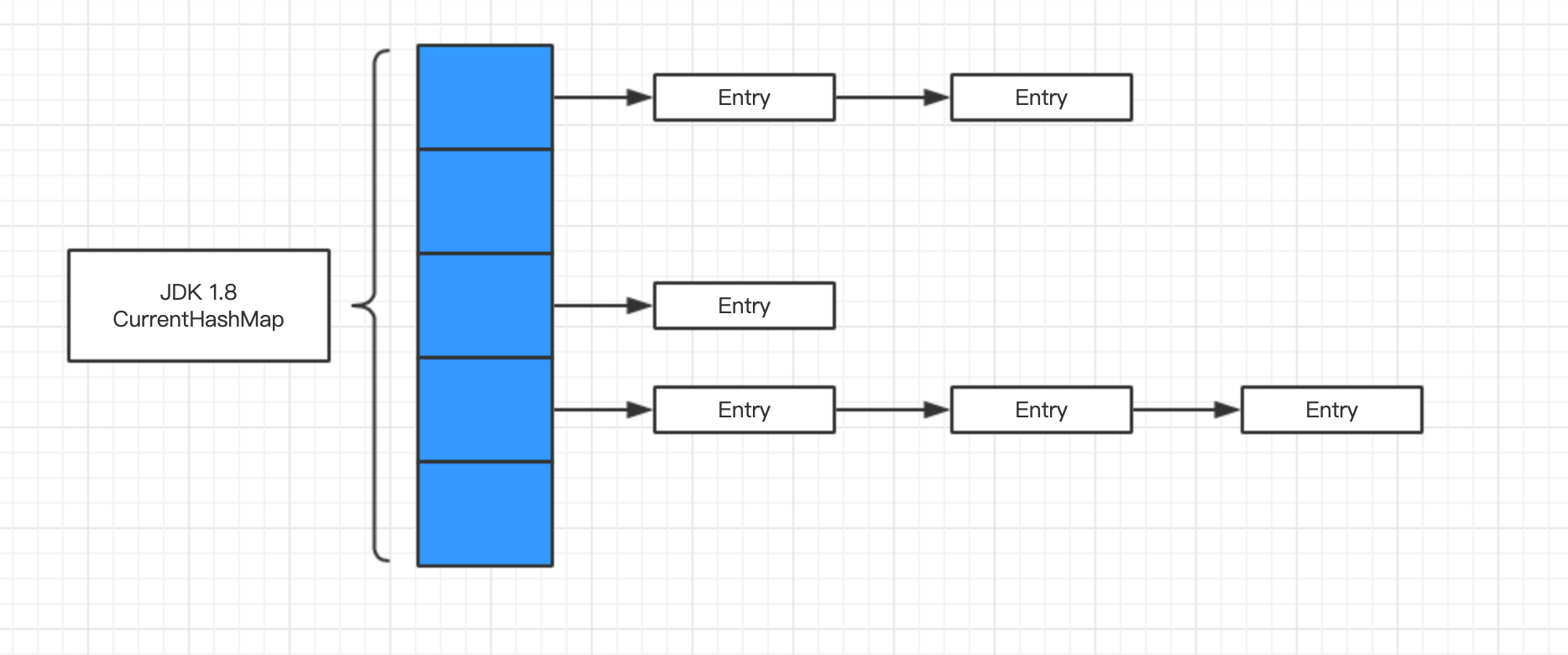version 1.7的时候根据Segment来给每一链配锁，但是带来的问题就是hash搜索时间变长。不过相较于Hashtable而言，性能上还是更加出色的。因为分段锁的机制也就不影响两两段之间并不会存在锁的问题，也就提高了性能。

# HashMap中的循环链

void transfer(Entry[] newTable){    Entry[] src = table;    int newCapacity = newTable.length;    // 很轻松是一个复制的工程    for (int j = 0; j < src.length; j++) {        Entry<K,V> e = src[j];        if (e != null) {            src[j] = null;            do {                Entry<K,V> next = e.next;                int i = indexFor(e.hash, newCapacity);                e.next = newTable[i];                newTable[i] = e;                e = next;            } while (e != null);        }    }}

## 单线程情况下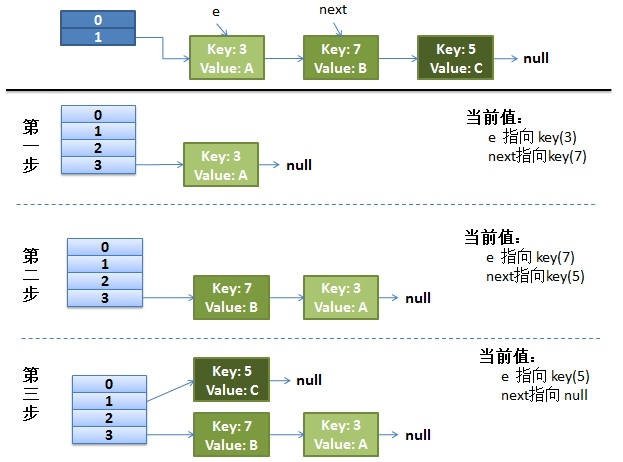## 高并发情况下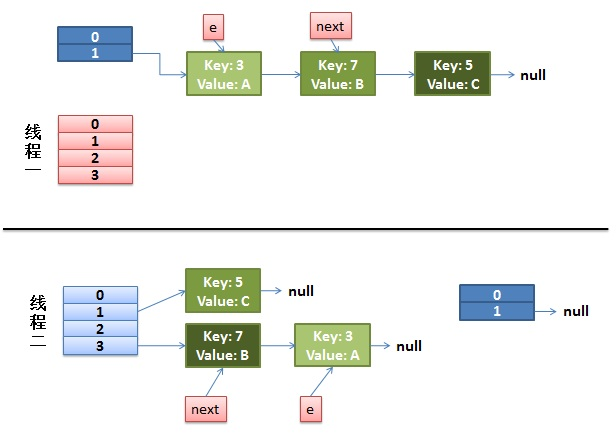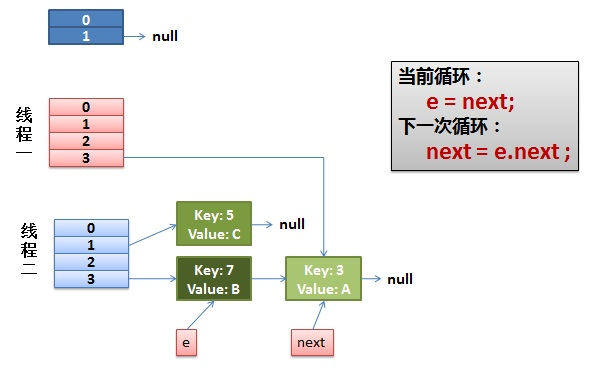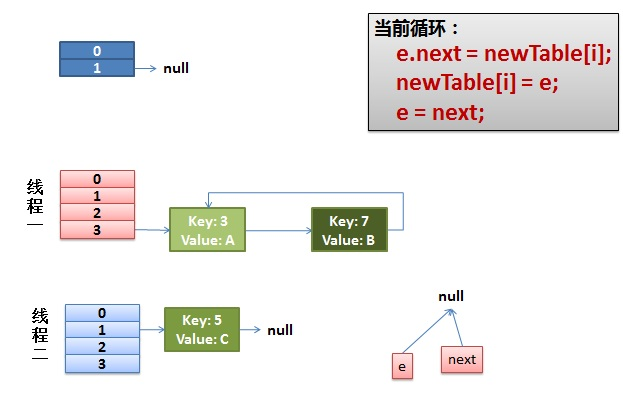# 总结## 评论